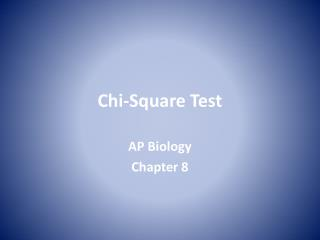DownloadDownload PresentationChi-Square Test

# Chi-Square Test

Download Presentation## Chi-Square Test

- - - - - - - - - - - - - - - - - - - - - - - - - - - E N D - - - - - - - - - - - - - - - - - - - - - - - - - - -
##### Presentation Transcript

1. Chi-Square Test AP Biology Chapter 8

2. Chi-Square Test • Random chance is a major determinant in the inheritance of alleles • Which alleles go to which gamete? • Which gamete combines with which gamete? • Could other factors or errors be influencing results? • Chance plays a major role determining which alleles • Biologists need a statistical tool which helps judge the probability that random chance influenced an outcome, such as a genetic cross.

3. Chi-Square Test • Chi-square test is that tool • Should there be a difference between observed results and predicted or expected results, Chi-square allows investigator to judge the influence of simple, random chance • If Chi-square indicates that the difference is likely not due to chance, then the investigator will know to look for other influencing factors

4. When using chi-square in biology, there is some vocabulary we must know: • Hypothesis = a proposed explanation of an observed phenomenon • Observed results= what you can observe during the course of an experiment • Expected results = what you expect to see based on your hypothesis (predictions)

5. Chi-Square Test • Hypothesis leads to certain prediction of data • Experiment results in observed data • The following equation calculates the likelihood that the difference is due to chance alone

6. Chi-Square Tutorial https://www.youtube.com/watch?v=WXPBoFDqNVk Paul Anderson, Bozeman Science View this tutorial before proceeding

7. Chi-Square Lab • Distribute • AP Lab Chi-Square Test by Foligia, 2004 • Students read lab carefully • two coins to each pair of students • Conduct lab

8. Free-Response Assessment Chapter 8 Free Response Question: Genes Are Particulate and Are Inherited According to Mendel’s Laws • Use a Chi-square test on the F2 generation data to analyze your predication of the parental genotypes. Show all your work and explain the importance of your final answer.